Line and Bar Plots¶

Tyler Caraza-Harter

Previously, we learned how to create matplotlib pie charts and scatter plots by calling Pandas plotting methods for Series and DataFrames.

In this document, we'll also learn how to also create line plots and bar plots.

Let's start by doing our matplotlib setup and usual imports:

In :
%matplotlib inline

In :
import pandas as pd
from pandas import Series, DataFrame


For readability, you may also want to increase the default font size at the start of your notebooks. You can do so by copy/pasting the following:

In :
import matplotlib
matplotlib.rcParams.update({'font.size': 15})


Line Plot from a Series¶

We can create a line plot from either a Series (with s.plot.line()) or a DataFrame (with df.plot.line()).

In :
s = Series([0,100,300,200,400])
s

Out:
0      0
1    100
2    300
3    200
4    400
dtype: int64
In :
s.plot.line()

Out:
<matplotlib.axes._subplots.AxesSubplot at 0x1118d6ba8>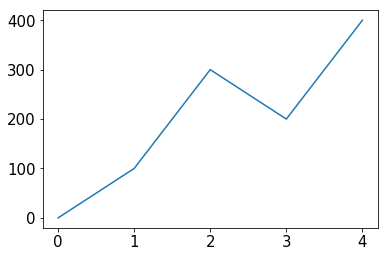The y values are clearly the values in the Series, but where are the x-values coming from? You guessed it, the Series' index. Let's try the same values with a different index.

In :
s = Series([0,100,300,200,400], index=[1,2,30,31,32])
s

Out:
1       0
2     100
30    300
31    200
32    400
dtype: int64
In :
s.plot.line()

Out:
<matplotlib.axes._subplots.AxesSubplot at 0x11cef6780>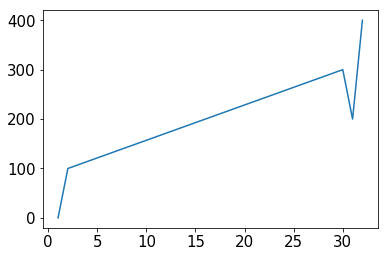Now we see that the plot starts from 1 (instead of 0) and a bigger gap in the index (between 2 and 30) corresponds to a bigger line segment over the x-axis.

What happens if our index is not in order?

In :
s = Series([0,100,300,200,400], index=[1,11,2,22,3])
s

Out:
1       0
11    100
2     300
22    200
3     400
dtype: int64
In :
s.plot.line()

Out:
<matplotlib.axes._subplots.AxesSubplot at 0x11cfab9e8>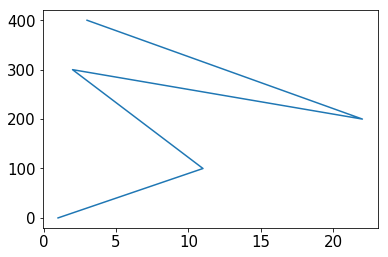Oops! That's probably not what we want. 99% of the time, people making a line plot want readers to be able to lookup a single y-value (per line) given a point along the x-axis. So even though this line passes through all of our data points, the lines between the points are very misleading.

If your data isn't already sorted, you'll probably want to sort it by the index first:

In :
s.sort_index()

Out:
1       0
2     300
3     400
11    100
22    200
dtype: int64

Don't get confused about this function! If we have a Python list L and we call L.sort(), the items in L are rearranged in place and the sort function doesn't return anything.

In contrast, if we have a Pandas Series s and we call s.sort_index(), the items in S are not moved, but the sort_index function returns a new Series that is sorted. So if we print s again, we see the original (unsorted) data:

In :
s

Out:
1       0
11    100
2     300
22    200
3     400
dtype: int64

Because sort_index() returns a new Series and we can call .plot.line() on a Series, we can do the following on an unsorted Series s in one step:

In :
s.sort_index().plot.line()

Out:
<matplotlib.axes._subplots.AxesSubplot at 0x11cf09860>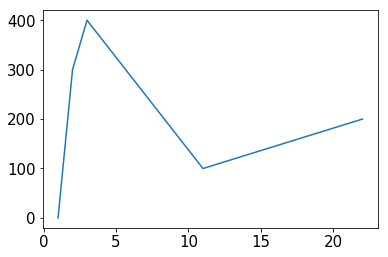Line Plot from a DataFrame¶

In addition to the Series.plot.line() method, there is also a DataFrame.plot.line() method. Whereas the line function for a Series creates a plot with a single line, the line plot for a DataFrame draws a line for each column in the DataFrame (remember that each column in a DataFrame is essentially just a Series).

Let's try with a DataFrame containing temperature patterns for Madison, WI. The data was copied from https://www.usclimatedata.com/climate/madison/wisconsin/united-states/uswi0411, and contains the typical daily highs and lows for each month of the year.

In :
df = DataFrame({
"high": [26, 31, 43, 57, 68, 78, 82, 79, 72, 59, 44, 30],
"low": [11, 15, 25, 36, 46, 56, 61, 59, 50, 39, 28, 16]
})

df

Out:
high low
0 26 11
1 31 15
2 43 25
3 57 36
4 68 46
5 78 56
6 82 61
7 79 59
8 72 50
9 59 39
10 44 28
11 30 16
In :
df.plot.line()

Out:
<matplotlib.axes._subplots.AxesSubplot at 0x11d1e0c50>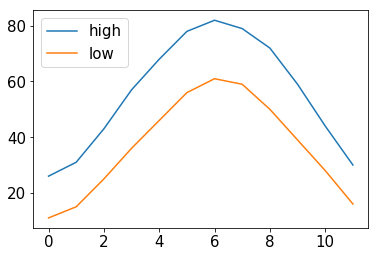Not bad! We can see the temperatures vary througout the year, with highs correlated with lows. But what is the x-axis? What is the y-axis?

Remember that calling an AxesSubplot object. There are AxesSubplot.set_xlabel and AxesSubplot.set_ylabel functions that will help us out here. Just to make sure to call them in the same cell where .plot.line is called, or the plot will be displayed before they can have an effect.

In :
ax = df.plot.line()
ax.set_xlabel('Month')
ax.set_ylabel('Temp (Fehrenheit)')

Out:
Text(0,0.5,'Temp (Fehrenheit)')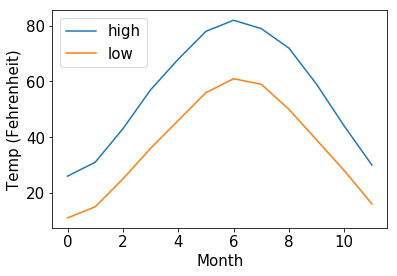What if we want the plot in Celcius? That's easy enough with some element-wise operations.

In :
c_df = DataFrame()
c_df["high"] = (df["high"] - 32) * (5/9)
c_df["low"] = (df["low"] - 32) * (5/9)
c_df

Out:
high low
0 -3.333333 -11.666667
1 -0.555556 -9.444444
2 6.111111 -3.888889
3 13.888889 2.222222
4 20.000000 7.777778
5 25.555556 13.333333
6 27.777778 16.111111
7 26.111111 15.000000
8 22.222222 10.000000
9 15.000000 3.888889
10 6.666667 -2.222222
11 -1.111111 -8.888889
In :
ax = c_df.plot.line()
ax.set_xlabel('Month')
ax.set_ylabel('Temp (Celsius)')

Out:
Text(0,0.5,'Temp (Celsius)')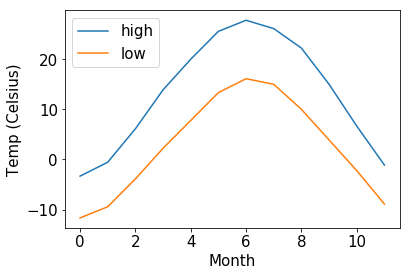That's looking good!

One small thing: did you notice the extra print above the plot that says Text(0,0.5,'Temp (Celsius)')? That happened because the call to set_ylabel returned that value. We could always put None at the end of our cell to supress that:

In :
ax = c_df.plot.line()
ax.set_xlabel('Month')
ax.set_ylabel('Temp (Celsius)')
NoneTick Labels¶

The above plot would be nicer if we saw actual month names along the y-axis. Let's create a DataFrame with the same data, but month names for the index.

In :
df = DataFrame({
"month": ["Jan", "Feb", "Mar", "Apr", "May", "Jun", "Jul", "Aug", "Sep", "Oct", "Nov", "Dec"],
"high": [26, 31, 43, 57, 68, 78, 82, 79, 72, 59, 44, 30],
"low": [11, 15, 25, 36, 46, 56, 61, 59, 50, 39, 28, 16]
})

df = df.set_index("month")


Out:
high low
month
Jan 26 11
Feb 31 15
Mar 43 25
Apr 57 36
May 68 46

Let's try plotting it.

In :
ax = df.plot.line()
ax.set_xlabel('Month')
ax.set_ylabel('Temp (Fehrenheit)')
None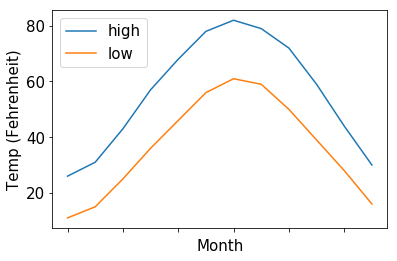Unfortunately, even though we now have months for the index, matplotlib won't use them for the x-axis unless we specifically tell it to. We can explicitly give matplotlib tick labels with the set_xticklabels method.

In :
# careful, this is an example of a bad plot!
ax = df.plot.line()
ax.set_xticklabels(df.index)
None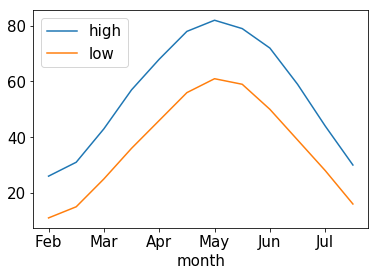Yikes! That's not what we wanted at all. The above plot starts at Feb (instead of Jan), and it only covers half a year. We've set the tick labels, but not the tick positions. Let's take a look at the positions:

In :
ax.get_xticks()

Out:
array([-2.,  0.,  2.,  4.,  6.,  8., 10., 12.])

You should read the above as follows:

• the first tick label (Jan) is drawn at position -2, which is out of the plots range (so we don't see Jan)
• the second tick label (Feb) is drawn at position 0 (the leftmost)
• the third tick label (Mar) is drawn at position 2
• and so on

Fortunately, we can set the tick positions explicitly. The only correct configuration in this case is 0, 1, 2, 3, ...

In :
ax = df.plot.line()
ax.set_xticks([0, 1, 2, 3])
ax.set_xticklabels(df.index)
None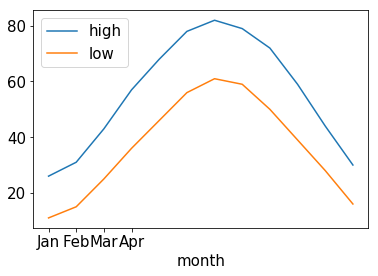If we want to count from 0 to 11, we can use range(len(df.index)).

In :
ax = df.plot.line()
ax.set_xticks(range(len(df.index)))
ax.set_xticklabels(df.index)
None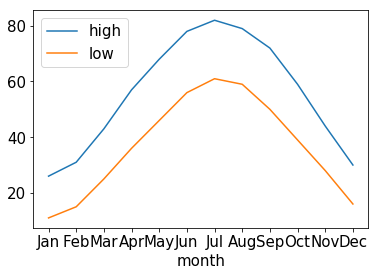This plot is correct, but crowded! There are two solutions: (1) make the plot wider or (2) rotate the labels. We'll demo both. We'll also add back the axis labels.

In :
# approach 1: wider plot
ax = df.plot.line(figsize=(8,4)) # this is the (width,height)
ax.set_xticks(range(len(df.index)))
ax.set_xticklabels(df.index)
ax.set_xlabel('Month')
ax.set_ylabel('Temp (Fehrenheit)')
None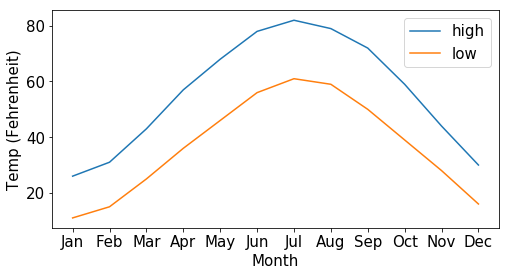In :
# approach 2: rotate ticks
ax = df.plot.line()
ax.set_xticks(range(len(df.index)))
ax.set_xticklabels(df.index, rotation=90) # 90 is in degrees
ax.set_xlabel('Month')
ax.set_ylabel('Temp (Fehrenheit)')
None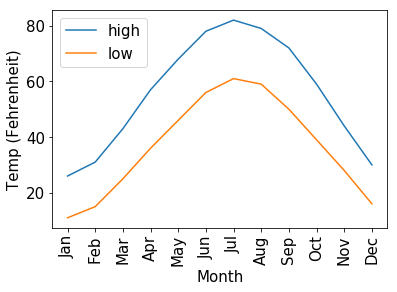Example: Stock Market Returns¶

In this example, we'll plot the performance of American stocks from 1970 to 2017. Specifically, we'll be looking at S&P 500 index data. The S&P 500 index tracks how well the 500 largest public American companies are collectively worth (think of it as a weighted average with more valuable companies being weighted more heavily).

We'll get our data from the Wikipedia on the S&P 500 Index article. Take a moment to skip the article.

We're interested in the "Total Annual Return Including Dividends" column of the table in the "Annual returns" section. Investors make money when (1) stock prices rise, or (2) companies pay dividends to shareholders. This column captures the yearly return, considering both these factors.

There are two three in this example. First, we do some web scraping to collect the data (it's a details BeautifulSoup example). While this section may be useful to you in the future, you won't need to know BeautifulSoup for P10 or the final exam, so feel free to skip to part 2 if this section doesn't interest you. For part 2, we'll visualise the data in several ways. In part 3, we'll simulate stock market returns, sampling from the real data in order to explore possible investment outcomes.

Stock Market Part 1: Collecting the Data¶

As a first step, let's download the wiki page and save it to a file named sp500.html. We check if this file exists before doing the download. If it does, we just use the contents of sp500.html instead of fetching the data again from Wikipedia (it's faster to access data on your computer rather than from a website).

In :
import os, requests

path = "sp500.html"

if not os.path.exists(path):
r = requests.get('https://en.wikipedia.org/wiki/S%26P_500_Index')
r.raise_for_status()
f = open(path, "w")
f.write(r.text)
f.close()

f = open(path)
f.close()

In :
# let's parse the HTML
from bs4 import BeautifulSoup
page = BeautifulSoup(html, 'html.parser')


The page contains six tables. Which one has the data we care about? We can loop over each table, convert the contents to text, and check with the text contains the term "Total Annual Return" (that's the name of the column with the data we want).

In :
target_column = "Total Annual Return"
tab = None
for curr in page.find_all('table'):
if curr.get_text().find(target_column) >= 0:
tab = curr
break
assert(tab != None)


Now we have the table we want. Let's create a list of lists representing the table data. This will be a list of rows, where each row contains td (table data) and th (table header) elements. Both of these elements are used to represent cells in HTML tables.

In :
rows = []
for tr in tab.find_all('tr'):
rows.append(tr.find_all(['td', 'th']))

# let's print the first three rows to make sure they are what we expect.
rows[:3]

Out:
[[<th>Year
</th>, <th>Change in Index
</th>, <th>Total Annual Return Including Dividends
</th>, <th>Value of 1.00 Invested on 1970‑01‑01 </th>, <th>5 Year Annualized Return </th>, <th>10 Year Annualized Return </th>, <th>15 Year Annualized Return </th>, <th>20 Year Annualized Return </th>, <th>25 Year Annualized Return </th>], [<td>1970 </td>, <td align="right">0.10% </td>, <td align="right">4.01% </td>, <td align="right">1.04
</td>, <td align="right">-
</td>, <td align="right">-
</td>, <td align="right">-
</td>, <td align="right">-
</td>, <td align="right">-
</td>], [<td>1971
</td>, <td align="right">10.79%
</td>, <td align="right">14.31%
</td>, <td align="right">1.19 </td>, <td align="right">- </td>, <td align="right">- </td>, <td align="right">- </td>, <td align="right">- </td>, <td align="right">- </td>]] Let's make sure (with asserts) that the 0th and 2nd columns contain year and annual return data. If they do, we want to extract these entries and construct a Series with year as index and annual return for values. In : assert(rows.get_text().find("Year") >= 0) assert(rows.get_text().find("Total Annual Return") >= 0) index = [] values = [] for row in rows[1:]: index.append(row.get_text().strip()) values.append(row.get_text().strip()) if index[-1] == '2017': break returns = Series(values, index=index) returns.head()  Out: 1970 4.01% 1971 14.31% 1972 18.98% 1973 −14.66% 1974 −26.47% dtype: object Let's normalize the data so we can use it to multiply initial money. For example, we want to convert 4% to 1.04. That way, if we start with \100, we can multiply by 1.04 to compute that we have $104 after a year. Don't worry about the replace of chr(8722). It's not important to the example. In : print("'{}' is a weird dash, not the negative dash '-' that will let us convert to a float.".format(chr(8722))) mults = returns.str.replace(chr(8722), "-").str.replace("%", "").astype(float) / 100 + 1 mults.head()  '−' is a weird dash, not the negative dash '-' that will let us convert to a float.  Out: 1970 1.0401 1971 1.1431 1972 1.1898 1973 0.8534 1974 0.7353 dtype: float64 We'll save this nicely formatted data to a CSV file. Any analysis of returns can use that directly without needing to repeat this HTML parsing. In : df = DataFrame({"year":mults.index, "return":mults.values}) df.to_csv("sp500.csv", index=False) df.head()  Out: year return 0 1970 1.0401 1 1971 1.1431 2 1972 1.1898 3 1973 0.8534 4 1974 0.7353 Stock Market Part 2: Plotting¶ In the previous step, we generated sp500.csv. Let's read that in and start doing some plotting. There are a few things we want to plot: • returns each year • total returns over time • correlation between the returns in one year and the subsequent year In : df = pd.read_csv("sp500.csv") df.head()  Out: year return 0 1970 1.0401 1 1971 1.1431 2 1972 1.1898 3 1973 0.8534 4 1974 0.7353 Lets use the year as the index. In : df = df.set_index("year") df.head()  Out: return year 1970 1.0401 1971 1.1431 1972 1.1898 1973 0.8534 1974 0.7353 Plot 1: returns each year. We want the year for the x-axis and the return on the y-axis. In : df.plot.line()  Out: <matplotlib.axes._subplots.AxesSubplot at 0x1202088d0>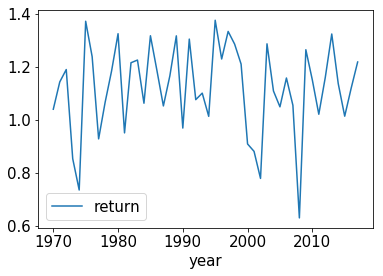We see a lot of noise, but the line stays above 1 in most years. Plot 2: total returns over time. The x-axis will be time, and the y-axis will be total returns. We will assume we started in 1970 with \$1000.

In order to get the total money in a given year, we want to multiply the starting money by all the return multiples up through that year (this is called a compounding return). We can use the cumprod method for this.

In :
df['return'].cumprod().head()

Out:
year
1970    1.040100
1971    1.188938
1972    1.414599
1973    1.207219
1974    0.887668
Name: return, dtype: float64

For example, the 1973 value of 1.207 came by multiplying 1.0401 * 1.1431 * 1.1898 * 0.8534 (the multiples for 1970 through 1973). Let's plot how much money we have over time, if we start with $1000. In : total = 1000 * df['return'].cumprod() total.head()  Out: year 1970 1040.100000 1971 1188.938310 1972 1414.598801 1973 1207.218617 1974 887.667849 Name: return, dtype: float64 In : ax = total.plot.line() ax.set_ylabel('Net Worth') None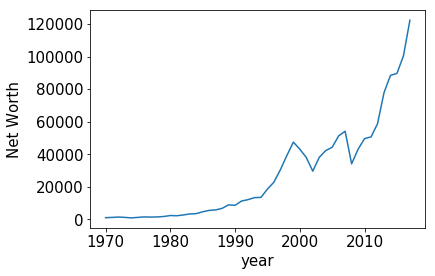Plot 3: do a scatter to show the correlation between one year and the next. To do this, we'll create two Series, both indexed by year. The first Series we'll pull directly from sp500.csv: the index will be a year, and the corresponding value will be the returns for that year. In the second Series, the index will be a year, and the value will the the returns in the year FOLLOWING the year in the index. In : df = pd.read_csv("sp500.csv") df.head()  Out: year return 0 1970 1.0401 1 1971 1.1431 2 1972 1.1898 3 1973 0.8534 4 1974 0.7353 In : df = df.set_index("year") df.head()  Out: return year 1970 1.0401 1971 1.1431 1972 1.1898 1973 0.8534 1974 0.7353 In : series1 = df['return'] series2 = Series(df['return'].values[1:], index=df['return'].index[:-1]) pairs = DataFrame({"curr":series1, "next":series2}) pairs.head()  Out: curr next year 1970 1.0401 1.1431 1971 1.1431 1.1898 1972 1.1898 0.8534 1973 0.8534 0.7353 1974 0.7353 1.3720 As you can see, the next column of the 1970 year contains the curr value of the 1971 year. Let's do a scatter plot to look at the correlation. As a pre-step, we'll subtract 1 from ever cell so a 10% loss will be represented as -0.1 (instead of 0.9). In : (pairs - 1).head()  Out: curr next year 1970 0.0401 0.1431 1971 0.1431 0.1898 1972 0.1898 -0.1466 1973 -0.1466 -0.2647 1974 -0.2647 0.3720 In : (pairs - 1).plot.scatter(x='curr', y='next')  Out: <matplotlib.axes._subplots.AxesSubplot at 0x120340160>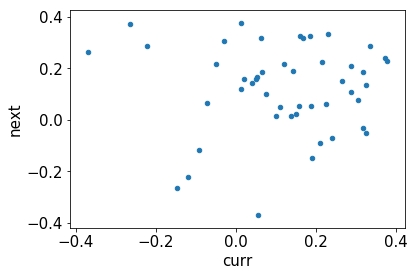Stock Market Part 3: Simulation¶ In this section, we'll going explore likely outcomes if one were to invest \$1000 in an S&P 500 index fund for 10 years.

In :
df = pd.read_csv("sp500.csv")

Out:
year return
0 1970 1.0401
1 1971 1.1431
2 1972 1.1898
3 1973 0.8534
4 1974 0.7353
In :
returns = df['return']

Out:
0    1.0401
1    1.1431
2    1.1898
3    0.8534
4    0.7353
Name: return, dtype: float64
In :
import random
sim = DataFrame()

# do 25 simulations
for i in range(25):
# sample returns for 10 years

# the course of the decade

# add this simulation as a column in the DataFrame
sim['sim'+str(i)] = net_worth

sim

Out:
sim0 sim1 sim2 sim3 sim4 sim5 sim6 sim7 sim8 sim9 ... sim15 sim16 sim17 sim18 sim19 sim20 sim21 sim22 sim23 sim24
0 909.000000 1285.800000 1323.900000 928.200000 1049.100000 1100.800000 1021.100000 1225.600000 735.300000 1375.800000 ... 1136.900000 969.000000 1304.700000 1049.100000 1286.800000 1317.300000 1062.700000 1062.700000 1189.800000 1150.600000
1 1107.434700 1337.360580 1602.448560 1101.494940 1368.760770 1161.233920 1291.283060 1451.600640 627.505020 1672.284900 ... 1152.589220 1172.877600 1373.196750 1388.903490 1133.799480 1403.714880 1121.042230 1462.062660 1566.847620 1067.986920
2 1274.214366 1761.170148 2123.244342 1116.695570 1435.966924 1300.117497 1495.176655 1921.774087 746.605473 1760.079857 ... 1365.126672 862.416899 1463.278457 1905.575588 1248.086468 1849.113611 1388.298698 1907.553153 1665.088966 1120.425078
3 1753.064124 2264.512576 2796.949772 1295.366861 1745.417796 1712.124732 1922.498143 2544.236714 795.582792 2264.870760 ... 1393.930845 759.875530 1535.125429 2030.581347 1478.233612 2200.075375 1020.816032 2412.291717 1791.968745 1179.247394
4 1962.730594 2804.372374 2943.789635 1472.702585 2121.555331 2255.381909 1999.590319 3019.245709 1023.755937 2682.512929 ... 1846.958370 1006.835077 2112.025565 2142.060263 1723.768215 2724.573344 1182.002884 2793.192579 2203.404769 1256.606023
5 1443.195806 3188.290952 2982.647658 1550.019470 1810.535319 3094.383979 2476.292651 3249.312232 1037.883768 2550.533292 ... 2197.511068 1332.948959 2364.623823 2484.789905 1638.958819 3505.980979 1566.153821 2175.897019 2835.341257 1318.305379
6 1501.067957 3691.722094 3539.507976 2067.105966 1848.737615 3761.223727 2575.591986 4177.965668 1203.945171 2974.176872 ... 2498.350333 1351.343654 2862.140675 2407.761418 1444.086615 3725.805987 1854.952586 2524.040542 3051.394260 1516.842169
7 1393.291278 4304.917133 4383.326677 1821.327066 2377.106825 3967.714909 2629.936977 5090.015573 1027.446809 3297.767316 ... 2898.086387 1646.341974 3769.153055 2525.982503 1232.383518 2347.257772 2201.272234 2995.278911 3251.565724 1950.355661
8 1693.545548 5699.279793 5543.154916 2167.014944 2616.719193 4227.997007 2317.237470 6028.614444 1094.847320 3905.875609 ... 3448.143183 2195.561657 5026.542514 2945.548197 1462.469520 3107.534564 2092.969640 3854.324903 3998.125214 2600.994310
9 2082.383606 4863.765375 4318.117679 2477.114782 3467.152931 5638.456809 2349.215347 6636.298780 1325.203196 3441.466999 ... 4226.044285 2087.540023 5341.706730 4041.292127 1950.349352 4093.555281 2120.596839 4874.179272 4570.256932 3221.071353

10 rows × 25 columns

Each of the above columns in the above DataFrame represents a simulation. The bottom row represents the total wealth after 10 years.

Let's plot each simulation. We'll disable the legend because 25 legend entries is too many.

In :
sim.plot.line(legend=False, figsize=(8,8))

Out:
<matplotlib.axes._subplots.AxesSubplot at 0x120427400>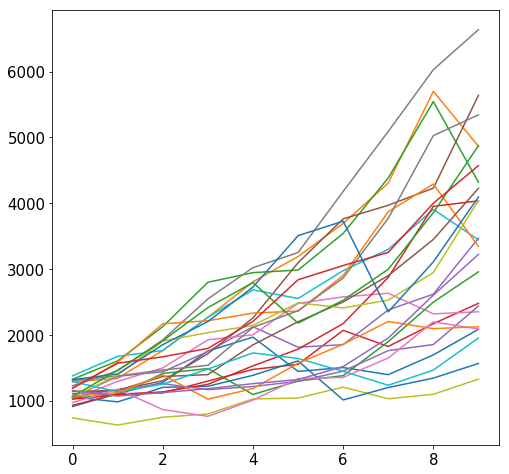It appears that doubling one's money (or better) over 10 years is fairly like. Of course, in some cases wealth increases very little (or worse, decreases). We also observe that the road to wealth is usually bumpy.

Bar Plots¶

Just like a line plot, bar plots can be created from either a Pandas Series or DataFrame. For our example data, let's learn a bit about the fire hydrants around the city of Madison. Data describing each fire hydrant can be found at http://data-cityofmadison.opendata.arcgis.com/datasets/54c4877f16084409849ebd5385e2ee27_6. We have already downloaded the data to a file named "Fire_Hydrants.csv". Let's read it and preview a few rows.

In :
df = pd.read_csv('Fire_Hydrants.csv')

Out:
X Y OBJECTID CreatedBy CreatedDate LastEditor LastUpdate FacilityID DataSource ProjectNumber ... Elevation Manufacturer Style year_manufactured BarrelDiameter SeatDiameter Comments nozzle_color MaintainedBy InstallType
0 -89.519573 43.049308 2536 NaN NaN WUJAG 2018-06-07T19:45:53.000Z HYDR-2360-2 FASB NaN ... 1138.0 NaN Pacer 1996.0 5.0 NaN NaN blue MADISON WATER UTILITY NaN
1 -89.521988 43.049193 2537 NaN NaN WUJAG 2018-06-07T19:45:53.000Z HYDR-2360-4 FASB NaN ... 1170.0 NaN Pacer 1995.0 5.0 NaN NaN blue MADISON WATER UTILITY NaN
2 -89.522093 43.048233 2538 NaN NaN WUJAG 2018-06-07T19:45:53.000Z HYDR-2361-19 FASB NaN ... 1179.0 NaN Pacer 1996.0 5.0 NaN NaN blue MADISON WATER UTILITY NaN
3 -89.521013 43.049033 2539 NaN NaN WUJAG 2018-06-07T19:45:53.000Z HYDR-2360-3 FASB NaN ... 1163.0 NaN Pacer 1995.0 5.0 NaN NaN blue MADISON WATER UTILITY NaN
4 -89.524782 43.056263 2540 NaN NaN WUPTB 2017-08-31T16:19:46.000Z HYDR-2257-5 NaN NaN ... 1065.0 NaN Pacer 1996.0 5.0 NaN NaN blue MADISON WATER UTILITY NaN

5 rows × 25 columns

For our first example, let's see what nozzle colors are most common. We can get a Series summarizing the data by first extracting the nozzle_color column, then using the Series.value_counts() function to produce a summary Series.

In :
df['nozzle_color'].head()

Out:
0    blue
1    blue
2    blue
3    blue
4    blue
Name: nozzle_color, dtype: object
In :
df['nozzle_color'].value_counts()

Out:
blue      5810
Blue      1148
Green      320
Orange      74
BLUE        45
Red          9
green        9
orange       4
GREEN        1
ORANGE       1
white        1
C            1
Name: nozzle_color, dtype: int64

The above data means, for example, that there are 5810 "blue" nozzles and 1148 "Blue" nozzles. We can already see there is a lot of blue, but we would really like a total count, not confused by whether the letters are upper or lower case.

In :
df['nozzle_color'].str.upper().value_counts()

Out:
BLUE      7003
GREEN      330
ORANGE      79
RED          9
WHITE        1
C            1
Name: nozzle_color, dtype: int64

Great! It's not clear what "C" means, but the data is clean enough. Let's plot it with Series.plot.bar.

In :
counts = df['nozzle_color'].str.upper().value_counts()
counts.plot.bar()

Out:
<matplotlib.axes._subplots.AxesSubplot at 0x120688588>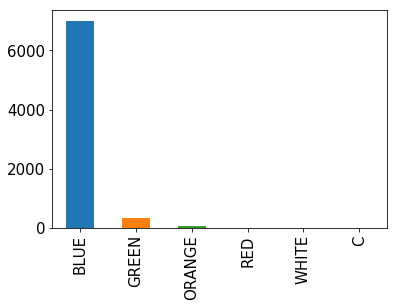Is the data reasonable? Try to notice next time you're walking by a hydrant. Consider it a challenge to spot a green nozzle (bonus points for orange!).

For our second question, let's create a similar plot that tells us what model of hydrants are most common. The model is represented by the Style column in the table. The following code is a copy/paste of above, just replacing "nozzle_color" with "Style":

In :
counts = df['Style'].str.upper().value_counts()
counts.plot.bar()

Out:
<matplotlib.axes._subplots.AxesSubplot at 0x120f865f8>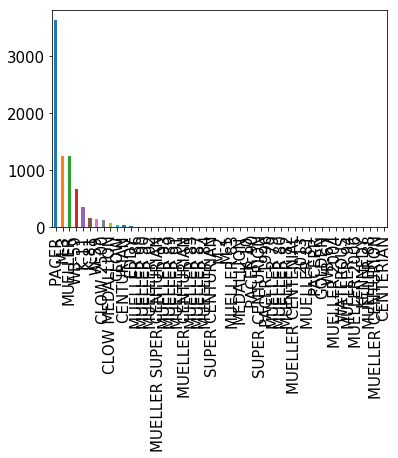Woah! That's way too much data. Let's just consider the top 10 models.

In :
top10 = counts[:10]
top10

Out:
PACER             3620
M-3               1251
MUELLER           1243
WB-59              664
K-11               351
K-81               162
W-59               151
CLOW 2500          123
CLOW MEDALLION      70
CLOW                50
Name: Style, dtype: int64

How many others are not in the top 10? We should show that in our results too.

In :
others = sum(counts[10:])
top10["others"] = others
top10

Out:
PACER             3620
M-3               1251
MUELLER           1243
WB-59              664
K-11               351
K-81               162
W-59               151
CLOW 2500          123
CLOW MEDALLION      70
CLOW                50
others             229
Name: Style, dtype: int64

Now that looks like what we want to plot.

In :
top10.plot.bar()

Out:
<matplotlib.axes._subplots.AxesSubplot at 0x1214d5b38>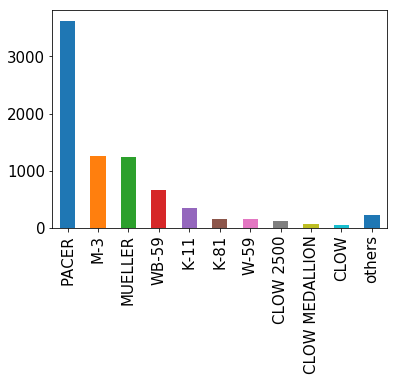Nice! This shows us what we want. We see Pacer is easily the most common. Some of the longer texts are harder to read vertically, so we also have the option to use .barh instead of .bar to rotate the bars.

In :
top10.plot.barh()

Out:
<matplotlib.axes._subplots.AxesSubplot at 0x11d754da0>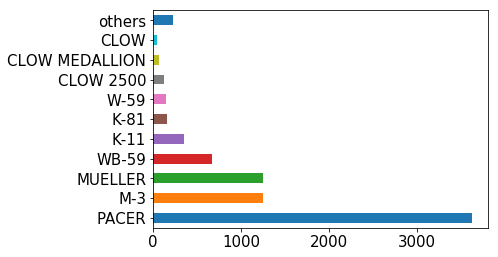I wonder what is up with all those Pacer hydrants? Have they always been so popular with the city? Turns out we can find out, because we also have a column called year_manufactured.

Let's find all the rows for Pacer hydrants and extract the year.

In :
pacer_years = df[df['Style'] == 'Pacer']['year_manufactured']

Out:
0    1996.0
1    1995.0
2    1996.0
3    1995.0
4    1996.0
Name: year_manufactured, dtype: float64

Let's round to the decade. We can do that by dividing by 10 (integer division), then multiplying by 10 again.

In :
pacer_decades = pacer_years // 10 * 10

Out:
0    1990.0
1    1990.0
2    1990.0
3    1990.0
4    1990.0
Name: year_manufactured, dtype: float64

How many Pacers were there each decade?

In :
pacer_decades.value_counts()

Out:
2000.0    1730
1990.0     846
2010.0     503
1980.0      21
1960.0       1
Name: year_manufactured, dtype: int64

Let's do the same thing in one step for non-pacers. That is, we'll identify non-pacers, extract the year, round to the decade, and then count how many entries there are per decade.

In :
other_decades = df[df['Style'] != 'Pacer']['year_manufactured'] // 10 * 10

Out:
2010.0    1196
1980.0     937
1970.0     578
1990.0     431
1950.0     371
1960.0     349
2000.0     215
1940.0      68
1930.0       9
1900.0       1
Name: year_manufactured, dtype: int64

Let's line up these two Series side-by-side in a DataFrame

In :
pacer_df = DataFrame({
})
pacer_df

Out:
pacer other
1900.0 NaN 1
1930.0 NaN 9
1940.0 NaN 68
1950.0 NaN 371
1960.0 1.0 349
1970.0 NaN 578
1980.0 21.0 937
1990.0 846.0 431
2000.0 1730.0 215
2010.0 503.0 1196

That looks plottable!

In :
pacer_df.plot.bar()

Out:
<matplotlib.axes._subplots.AxesSubplot at 0x1215b6320>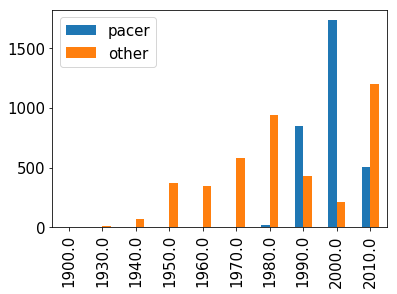That plot shows that the city started getting Pacers in the 90's. Most were from the 2000 decade, and it seems there is finally a shift to other styles.

While this plot is fine, when multiple bars represent a breakdown of a total amount, it's more intuitive to stack the bars over each other. This is easy with the stacked= argument.

In :
pacer_df.plot.bar(stacked=True)

Out:
<matplotlib.axes._subplots.AxesSubplot at 0x121db94e0>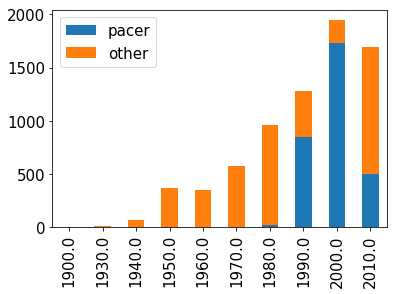This data supports all the same conclusions as before, and now one more thing is obvious: although there was stead growth in the number of hydrants over several decades, things seem to have leveled off more recently. Why? Further probing of the data might provide an answer. One explanation is that the 2000 decade contains 10 years, but we have a couple years left for the 10's. Perhaps this decade will still catch up.

Conclusion¶

After this reading, you should now be ready to create four types of plots: pie charts, scatter plots, line plots, and bar plots.

We saw that both line and bar plots can be created from either a single Series or a DataFrame. When created from a single Series, we end up with either a single line (for a line plot) or one set of bars (for a bar plot).

When we create from a DataFrame, we get multiple lines (one per column) for a line plot. And for a bar plot, we get multiple sets of bars. We can control whether those bars are vertical (with .bar) or horizontal (with .barh), as well as whether the bars are stacked or side-by-side.